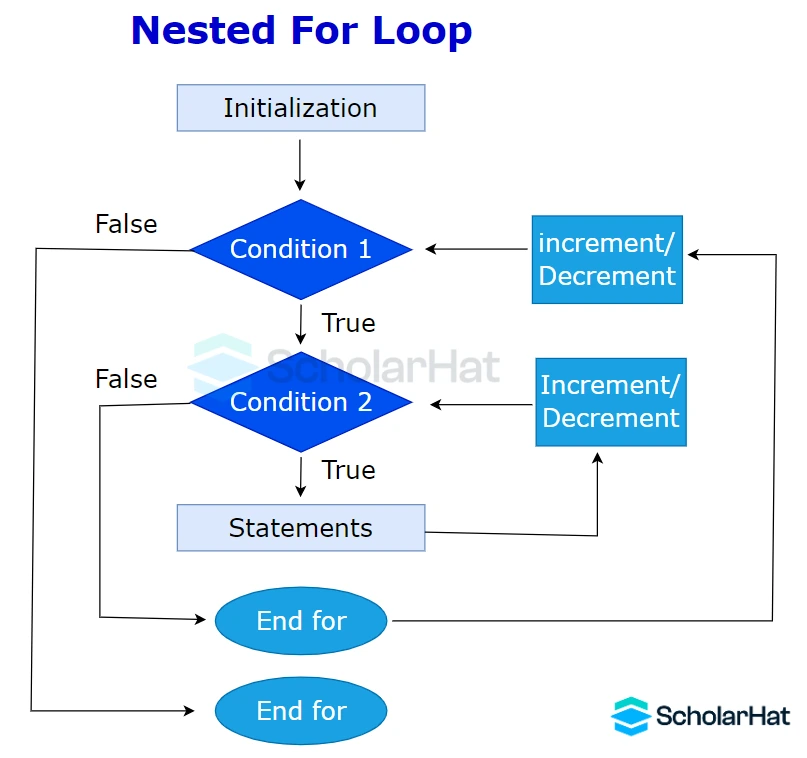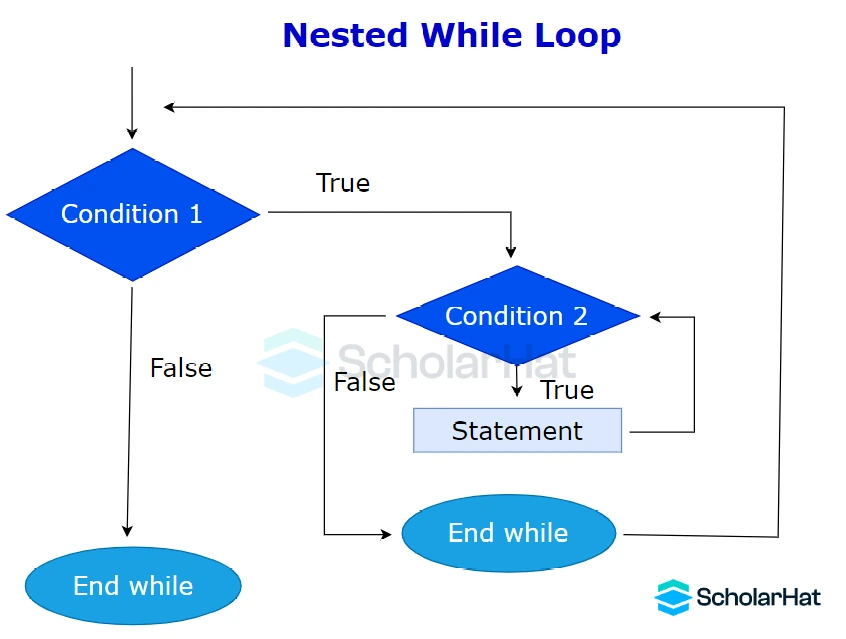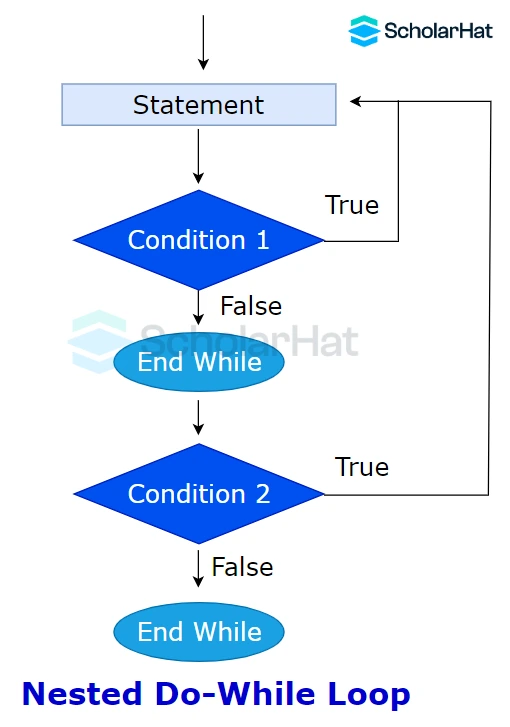# Nested Loops in C - Types of Expressions in C ( With Examples )

04 Dec 2023
Beginner
5.05K ViewsLearn via Video Course & by Doing Hands-on Labs

## Nested Loops in C: An Overview

Do you study C programming? For efficient repeating activities and handling complicated algorithms, nested loops are essential. Understanding how these work in C for beginners will save your time and ensure error-free code. In this article, we will dive deeper into these looping structures and provide valuable insights to assist you in your C training journey. We will discuss nested loops and infinite loops, their uses, and their implementation in the C programming language.

## What is the Nested loop in C?

Nested loops in C are a powerful programming concept that allows developers to implement complex iterations and repetitive tasks with greater efficiency. An inner loop can run repeatedly for each cycle of the outer loop when there is a nested loop, which is a loop inside another loop.

This structure is particularly useful when working with `multidimensional arrays` , intricate patterns, or scenarios that require repeated calculations. Code readability and maintainability are improved by nested loops in C.

#### Syntax

``````Outer_loop
{
Inner_loop
{
// inner loop statements.
}
// outer loop statements.
}
``````

## Use of nested loops in C

• When you need to do repeating operations inside of another loop,nested loops in C are helpful.
• They frequently handle matrices or multidimensional arrays.
• For iterating through intricate data structures like nested lists or trees, nested loops can also be used.
• Nesting loops are in handy when you need to conduct combinations or permutations of items.
• They are necessary for tackling issues like searching, sorting, or graph traversal that call for several iterations.
• When you need to compare elements from one loop with elements from another loop, use the nested loop in C programming to create more complex reasoning.

## Types of Nested loops in C

There are three types of nested loops in the C language

• Nested for loop in C
• Nested while loop in C
• Nested do-while loop in C

### Nested for loop in C

• The concept of the nested for loop in C programming language provides a versatile approach to achieve complex iterations and effectively execute repetitive tasks.
• In C, nested for loops make it easy to handle nested iterations, making jobs like browsing multidimensional arrays more simpler.
• This hierarchy enhances the readability, maintainability, and organization of the code.
• To tackle complex programming problems and investigate new avenues in software development, mastery is necessary.#### Example of nested for loop in C

``````
#include <stdio.h>
int main() {
int i, j;
for (i = 1; i <= 5; i++) {
for (j = 1; j <= i; j++) {
printf("%d ", j);
}
printf("\n");
}
return 0;
}``````

This C code generates a pattern of numbers using stacked loops. The inner loop (j) iterates from 1 to the current value of i, printing numbers and spaces, while the outer loop (i) iterates from 1 to 5, regulating the number of rows. As a result, there is a pattern of numerals rising in each row, with a newline character between each row.

#### Output

``````1
1 2
1 2 3
1 2 3 4
1 2 3 4 5
``````

### Nested while loop in C

• The nested while loop in C programming offers immense control and flexibility when solving multifaceted problems by allowing a programmer to place one loop inside another.
• Create complicated patterns and analyze data more easily with this framework.
• As a programmer, mastering the nested while loop in C will greatly expand the ability to handle challenging situations and develop intricate solutions.
• Dive into the world of C programming, and the user will find the nested while loop to be an invaluable tool in optimizing and streamlining the code to achieve extraordinary results.#### Example of nested while oop in C

``````
#include <stdio.h>
int main() {
int i = 1, j = 1;
while (i <= 3) {
printf("Outer loop iteration %d\n", i);
while (j <= 3) {
printf(" Inner loop iteration %d\n", j);
j++;
}
j = 1; // reset j to 1 for the next iteration of the outer loop
i++;
}
return 0;
}``````

Nesting while loops are present in this C code. The inner loop (j) iterates within each iteration of the outer loop (i), printing "Inner loop iteration" messages, while the outer loop (i) iterates three times, printing "Outer loop iteration" messages. After each inner loop is finished, j is reset to 1, resulting in nested iterations.

#### Output

``````Outer loop iteration 1
Inner loop iteration 1
Inner loop iteration 2
Inner loop iteration 3
Outer loop iteration 2
Inner loop iteration 1
Inner loop iteration 2
Inner loop iteration 3
Outer loop iteration 3
Inner loop iteration 1
Inner loop iteration 2
Inner loop iteration 3
``````

### Nested do...while loop in C

• The nested do-while loop in C programming serves as a powerful tool that grants coders the ability to efficiently execute repeated actions based on specific conditions.
• By executing commands while requirements are met, nested while loops improve precision as well as control in complex computations.
• As the developer dives deeper into the world of C programming, mastering the art of nested do-while loops will undoubtedly enhance the ability to create dynamic and effective solutions.#### Example of nested do...while loop in C

``````
#include <stdio.h>
int main() {
int i = 1, j;
do {
j = 1;
do {
printf("%d * %d = %d\n", i, j, i*j);
j++;
} while (j <= 10);
i++;
} while (i <= 2);
return 0;
}``````

This C code creates and outputs multiplication tables for the numbers 1 and 2 from 1 to 10 using a nested loop structure. It iterates over the values of 'i' (1 and 2) and 'j' (1 to 10), outputting the result of 'i * j' after each iteration using two nested "do-while" loops.

#### Output

``````1 * 1 = 1
1 * 2 = 2
1 * 3 = 3
1 * 4 = 4
1 * 5 = 5
1 * 6 = 6
1 * 7 = 7
1 * 8 = 8
1 * 9 = 9
1 * 10 = 10
2 * 1 = 2
2 * 2 = 4
2 * 3 = 6
2 * 4 = 8
2 * 5 = 10
2 * 6 = 12
2 * 7 = 14
2 * 8 = 16
2 * 9 = 18
2 * 10 = 20
``````

## FAQs

### 1. What is a nested loop?

A loop inside another loop is referred to as nested. It enables you to build a loop structure inside another loop by continually executing one or more statements in the body of the outer loop.

### 2. What are the advantages of nested loops?

The efficient handling of complicated data structures and multi-level iterative issues is made possible by nested loops. They are adaptable to different programming tasks.

### 3. What is a nested loop with an example?

Example: Print a multiplication table using stacked loops in which the inner loop iterates through the columns and the outer loop iterates through the rows.

### 4. What is the syntax of a nested loop?

The syntax often requires nesting loops, such as one "for" or "while" inside another "for" or "while." For example, in C:

``````for (int i = 0; i < 3; i++) {
for (int j = 0; j < 3; j++) {
// Inner loop statements
}
// Outer loop statements
}``````

### 5. What are three reasons to use nested loops?

Managing multi-dimensional data structures, addressing issues requiring several layers of iteration, and efficiently performing element combinations or permutations are three reasons to employ nested loops.

### 6. What are the 3 types of nested loops?

There aren't really "types" of nested loops; instead, they can be divided into groups according to what they're used for, such as nested "for" loops, nested "while" loops, or combinations of multiple loop types to meet varied programming requirements.

##### Summary
To conclude, nested loops are complex yet integral components of programming with C. Take your understanding of nested loops to the next level with a C Certification, ensuring you have the expertise to write optimized and error-free code. Understanding loop principles enables the selection of the appropriate loop for coding to be automatic. Despite their complexity, nested loops give programmers the freedom to design attractive solutions for more efficient complex procedures.
Share Article
Similar Articles